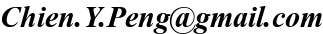• Version 2 and 3 differences in the input file: The input file has changed between Version 3 and Version 2. Parameters 8, 9, and 10 in Version 2 are now called 9, 10, and C0 in Version 3. C0 is now an optional parameter, so it is only visible when specified explicitly and given a value, just like Fourier modes, bending modes, spiral rotation functions, and truncation models.

• Three image header keywords that GALFIT looks for: EXPTIME, GAIN (or ATODGAIN), and NCOMBINE. If missing, the defaults are: EXPTIME=1, GAIN=7, NCOMBINE=1. Note that in Version 3, RDNOISE is no longer an important parameter unless one tells GALFIT to estimate a sigma-image by specifying a sky value manually (see the GALFIT manual for details). RDNOISE is now automatically estimated along with the background noise by GALFIT because for the sigma image it is not important to differentiate Poisson noise in the sky from detector RDNOISE.

Note: Sometimes an image may have units of [counts/sec] but the EXPTIME keyword does not show 1 second. This is misleading, but is unfortunately a common practice. Make sure that the EXPTIME keyword means what GALFIT expects: if the image is in flux units, EXPTIME = 1; if the image is in counts, EXPTIME should be the total exposure time; if the image is averaged over N images, each of which is T seconds, then NCOMBINE = N, and EXPTIME = T. Or else your magnitudes will be off by 2.5 log10(EXPTIME) mags.

• When one doesn't have an input sigma image: If an user doesn't provide a sigma image, or if one isn't found, GALFIT will create it internally. The sigma image is needed for estimating χ2. However, if you're letting GALFIT do this, be aware that the ADUs must be in COUNTS , not counts/sec. Furthermore, GALFIT needs to know how to convert from ADUs into electrons -- the meaningful unit for estimating Poisson noise -- via the GAIN of the CCD, and the NCOMBINE (the number of images averaged together) image header parameters in the image header. If GALFIT doesn't find these info, then it defaults to GAIN=7 and NCOMBINE=1, which are appropriate for WFPC2. Furthermore, if NCOMBINE is not 1, then GALFIT assumes that the image ADUs are averaged, rather than summed, over NCOMBINE number of images.

Note: for GALFIT to determine the sigma image correctly, the sky also ought to dominate the field of view.

• The correct way to fit a point source: The best way to fit a point source is to specify in Item D a PSF image (this is the same one used in convolution), then in the object list, specify ``psf'' for the object type. The free parameters for the PSF function are simply (x, y) and magnitude. GALFIT figures out the total magnitude by integrating over the PSF image and using the zeropoint in the input parameter file. Note that this method assumes the input PSF image contains 100% of the PSF flux. If this is not true, i.e. the PSF image cuts off the wings, then one would either need to make an aperture correction to account for the missing flux, or make the PSF image bigger.

This procedure for fitting a point source now differs from an earlier suggestion that the user should use a narrow Gaussian function, convolved with the PSF. Even though one can still do this it is not advisable because there likely will be numerical convergence issues, having to do with the gradient fitting within a single pixel, when the FWHM falls below 0.4 to 0.5 pixels. This may cause some or all of the parameters/components to be frozen. This technique, however, is still useful to test to see if an object is truly a point source. If one wants to do this, just make sure the FWHM does not dip below 0.4-0.5 pixels.

• How the PSF should be centered in the PSF image: If the PSF image has N=odd number of pixels along an axis, the centroid along that axis should be at the geometric center, i.e. N/2+0.5. If the PSF image has N=even number of pixels along an an axis, the centroid should be at pixel N/2+1. If the PSF is not centered as described, there will be a systematic offset in the measured position from actual. The offset is the same for all fitted components.

• Also, please see notes on Top 10 rules of thumb for galaxy fitting and Technical GALFIT FAQ .

Chien Peng ()